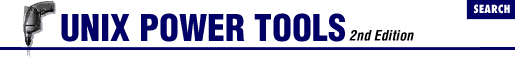home | O'Reilly's CD bookshelfs | FreeBSD | Linux | Cisco | Cisco Exam

#Chapter 45Shell Programming for the Initiated## 45.28 Quick Reference: expr

expr expr is a very handy tool in shell programming, since it provides the ability to evaluate a wide range of arithmetic, logical, and relational expressions. It evaluates its arguments as expressions and prints the result. expr is a standard UNIX utility; the GNU version is on the CD-ROM.

Here's the syntax. The [brackets] mean "optional"; don't type the brackets:

`expr` ```arg1 operator arg2 ``` `[` ```operator arg3 ``` `... ]`

Arguments and operators must be separated by spaces. In many cases, an argument is an integer, typed literally or represented by a shell variable. There are three types of operators: arithmetic, relational, and logical.

Exit status (44.7 ) values for expr are 0 if the expression evaluates non-zero and non-null, 1 if the expression evaluates to 0 or null, or 2 if the expression is invalid.

Arithmetic operators

Use these to produce mathematical expressions whose results are printed.

`+`

Add ```arg2 ``` to ```arg1 ``` .

`-`

Subtract ```arg2 ``` from ```arg1 ``` .

`*`

Multiply the arguments.

`/`

Divide ```arg1 ``` by ```arg2 ``` .

`%`

Take the remainder when ```arg1 ``` is divided by ```arg2 ``` .

Addition and subtraction are evaluated last, unless they are grouped inside parentheses. The symbols `*` , `(` , and `)` have meaning to the shell, so they must be escaped (preceded by a backslash or enclosed in quotes).

Relational operators

Use these to compare two arguments. Arguments can also be words, in which case comparisons assume a`<` z and A`<` Z. If the comparison statement is true, expr writes 1 to standard output (13.1 ) ; if false, it writes 0. The symbols `>` and `<` must be escaped.

`=`

Are the arguments equal?

`!=`

Are the arguments different?

`>`

Is ```arg1 ``` greater than ```arg2 ``` ?

`>=`

Is ```arg1 ``` greater than or equal to ```arg2 ``` ?

`<`

Is ```arg1 ``` less than ```arg2 ``` ?

`<=`

Is ```arg1 ``` less than or equal to ```arg2 ``` ?

Logical operators

Use these to compare two arguments. Depending on the values, the result written to standard output can be ```arg1 ``` (or some portion of it), ```arg2 ``` , or 0. The symbols `|` and `&` must be escaped.

`|`

Logical OR; if ```arg1 ``` has a non-zero (and non-null) value, the output is ```arg1 ``` ; otherwise, the output is ```arg2 ``` .

`&`

Logical AND; if both ```arg1 ``` and ```arg2 ``` have a non-zero (and non-null) value, the output is ```arg1 ``` ; otherwise, the output is 0.

`:`

Sort of like grep (27.1 ) ; ```arg2 ``` is a pattern to search for in ```arg1 ``` . ```arg2 ``` must be a regular expression in this case. If the ```arg2 ``` pattern is enclosed in `\( \)` , the output is the portion of ```arg1 ``` that matches; otherwise, the output is simply the number of characters that match. A pattern match always applies to the beginning of the argument (the `^` symbol is assumed by default).

### 45.28.1 Examples

Division happens first; output is 10:

```\$ ```expr 5 + 10 / 2
```
```

Addition happens first; output is 7 (truncated from 7.5):

```\$ ```expr \( 5 + 10 \) / 2
```
```

Add 1 to variable i ; this is how variables are incremented in Bourne shell scripts:

``````i=`expr "\$i" + 1`
```
```

Output 1 (true) if variable a is the string "hello":

```\$ ```expr "\$a" = hello
```
```

Output 1 (true) if variable b plus 5 equals 10 or more:

```\$ ```expr "\$b" + 5 \>= 10
```
```

In the examples below, variable p is the string "version.100". This command returns the number of characters in p :

```
\$ ```expr "\$p" : '.*'
```
Output is 11
```

Match all characters and print them:

```\$ ```expr "\$p" : '\(.*\)'
```
Output is "version.100"
```

Output the number of lowercase letters matched:

```\$ ```expr "\$p" : '[a-z]*'
```
Output is 7
```

Match a string of lowercase letters:

```\$ ```expr "\$p" : '\([a-z]*\)'
```
Output is \"version"
```

Truncate `\$x` if it contains five or more characters; if not, just output `\$x` . (Logical OR uses the second argument when the first one is 0 or null; i.e., when the match fails.)

```\$ ```expr "\$x" : '\(.....\)'  "\$x"
```
```

- DG from O'Reilly & Associates' UNIX in a Nutshell (SVR4/Solaris)45.27 Turn Off echo for "Secret" Answers45.29 Testing Characters in a String with expr